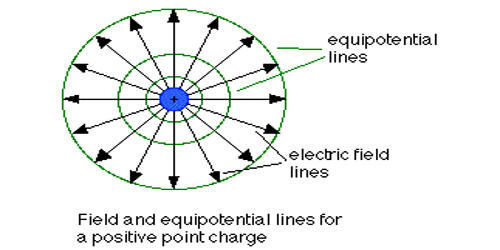Physics

# Explain Electric Potential of EarthEarth is an electric conductor. When a charged body is connected to the earth, it becomes electrically neutral. When a positively charged body is grounded electrons coming from the earth neutralize the body. Setting the potential of the Earth equal to zero is nothing more than a mathematical assumption for electrical engineering purposes. When a negatively charged body is grounded electrons from the body flow to the earth and the body becomes neutral. In reality, there is nothing such as an absolute potential. We only talk about the potential difference.

The earth is so big that if the charge is added or taken away from it its potential does not change at all. Likewise, if the water is taken away from sea or poured in the water level does not change. The earth is always taking charge from different bodies and simultaneously it supplies charge to other bodies. Hence earth is considered charge less. To determine the height of a place the height of the sea level is taken as zero, similarly to determine the potential of a body, the potential of the earth is taken as zero. Earth potential is taken as zero to make calculations easier, like zero deg cel temperature and zero bar gauge pressure.

Zero, Positive and Negative Potential:

Earth is not in zero potential, the earth is at lower potential compared to other potential sources found on earth. The potential of an uncharged conductor is taken as zero When a charged conductor is connected to the earth its potential becomes zero. Because, in the connected state, both the conductor and the earth is considered as a single conductor. The potential of a positively charged body is positive and negatively charged body is negative.

Earth is simply a commonly used reference point. Electric potential, the amount of work needed to move a unit charge from a reference point to a specific point against an electric field. The point-values of the potential are not observable. You can only observe differences in the potential. Typically, the reference point is Earth, although any point beyond the influence of the electric field charge can be used. You can for example always add a constant to the potential and have the same electric field, so that the potential is zero at a point can’t have physical significance.

However, to add to the confusion, the electrical potential of Earth is not the same everywhere. One end of your street could have an excess of electric charge with respect to the other end of your street, so if you grounded two different circuits on each end of your street and connected them together, you could end up with this charge flowing from one circuit to the other.